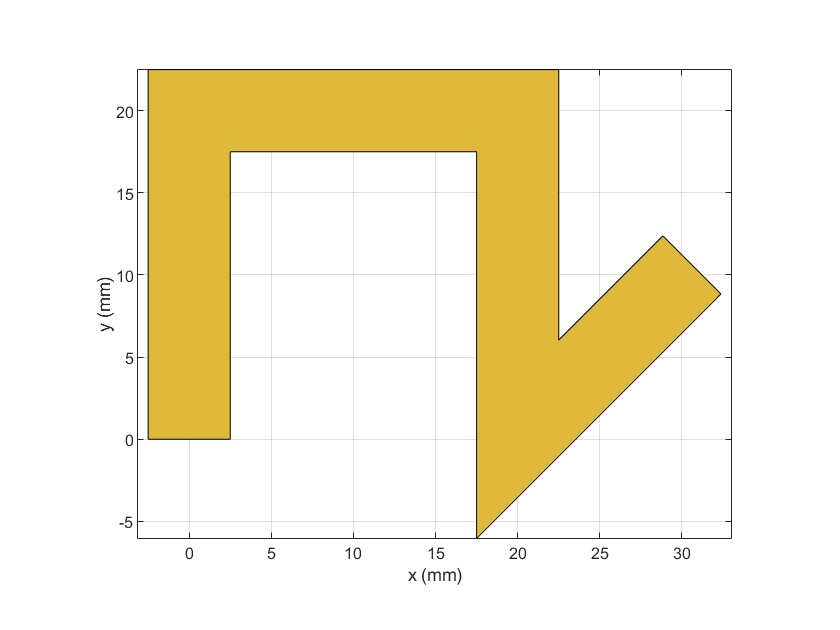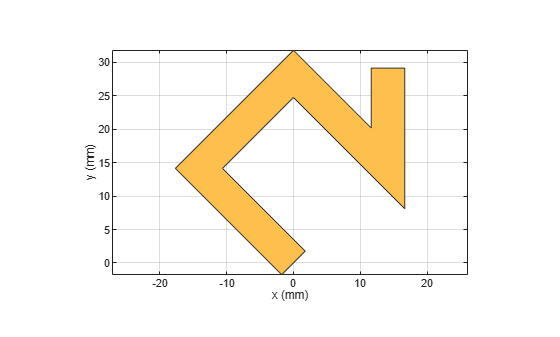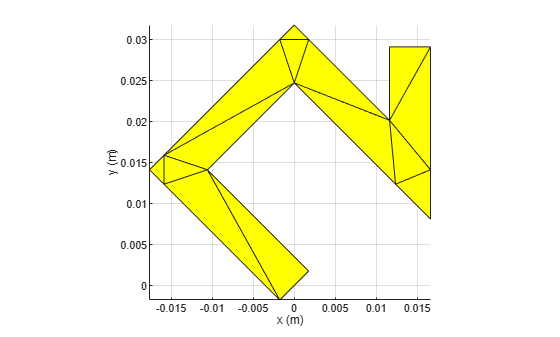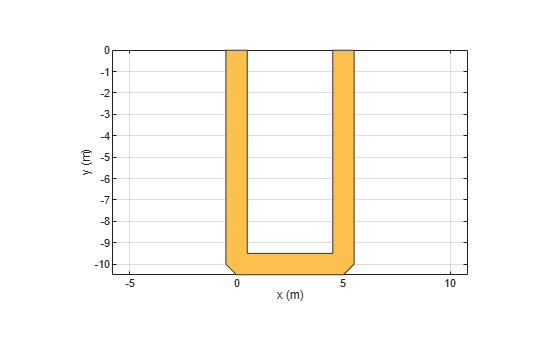traceLine

Create line trace

Description

Use the traceLine object to create a line trace. You can use this object to create lines of different lengths and different angles

Creation

Description

example

trace = traceLine creates a line trace using default properties.

example

trace = traceLine(Name=Value) sets properties using one or more name-value arguments. For example, traceLine('StartPoint',[1 1]) creates a line trace shape with the starting point of [1 1]. Properties not specified retain their default values.

Properties

expand all

Name of the line trace, specified as a character vector or string scalar.

Example: customtrace = traceLine(Name=traceline1)

Data Types: char | string

Start point of the line trace in Cartesian coordinates, specified as a two-element vector.

Example: customtrace = traceLine(StartPoint=[1 1])

Data Types: double

Length of line trace, specified as an n-by-1 vector in meters. Each element represents the length of a line segment.

Example: customtrace = traceLine(Length=[0.0100 0.0100 0.0100 0.0500])

Data Types: double

Width of the line trace, specified as a scalar or an n-by-1 vector in meters. Each element represents the length of a line segment

Example: customtrace = traceLine(Width=[0.0040 0.0040 0.0040 0.0050])

Data Types: double

Angle of the line trace, specified as an n-by-1 vector in degrees. Each element represents an angle of a line segment.

Example: customtrace = traceLine(Angle=[40 10 -40 35)

Data Types: double

Corner where two line segments interface, specified as either "Sharp", "Miter", or "Smooth". To apply the same value to all corners, specify a string scalar. For a different value for all corners, specify a (n-2)-by-1 vector of strings.

Example: trace = traceLine(Corner="Miter")

Data Types: string

Object Functions

 add Boolean unite operation on two RF PCB shapes and Shape1 & Shape2 for RF PCB shapes area Calculate area of RF PCB shape in square meters intersect Boolean intersection operation on two RF PCB shapes mesh Change and view mesh properties of metal or dielectric in PCB component minus Shape1 - Shape2 for RF PCB shapes plus Shape1 + Shape2 for RF PCB shapes rotate Rotate RF PCB shape about defined axis rotateX Rotate RF PCB shape about x-axis rotateY Rotate RF PCB shape about y-axis and angle rotateZ Rotate RF PCB shape about z-axis subtract Boolean subtraction operation on two RF PCB shapes scale Change size of RF PCB shape by fixed amount show Display PCB component structure or PCB shape translate Move RF PCB shape to new location

Examples

collapse all

Create a custom line trace with default properties.

customLine = traceLine
customLine =
traceLine with properties:

Name: 'mytraceLine'
StartPoint: [0 0]
Length: [0.0200 0.0200 0.0200 0.0150]
Width: 0.0050
Angle: [90 0 -90 45]
Corner: "Sharp"

View the trace.

show(customLine)Create a line trace.

customLine = traceLine;

Rotate the trace by 45 degrees along the Z-axis.

customLine = rotateZ(customLine,45)
customLine =
traceLine with properties:

Name: 'mytraceLine'
StartPoint: [0 0]
Length: [0.0200 0.0200 0.0200 0.0150]
Width: 0.0050
Angle: [90 0 -90 45]
Corner: "Sharp"

show(customLine)Mesh the line trace at a maximum edge length of 1 m.

mesh(customLine,MaxEdgeLength=1)Create and view a U-shaped line trace with mitered bends and a width of 1 m.

Ushapeline = traceLine;
Ushapeline.Length = [10 5 10];
Ushapeline.Angle  = [-90 0 90];
Ushapeline.Width  = 1;
Ushapeline.Corner = 2;
show(Ushapeline);